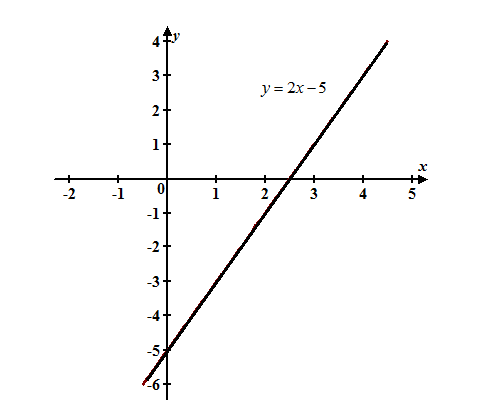# Comparing the algebraic and graphical methods for solving equations.### Precalculus: Mathematics for Calcu...

6th Edition
Stewart + 5 others
Publisher: Cengage Learning
ISBN: 9780840068071### Precalculus: Mathematics for Calcu...

6th Edition
Stewart + 5 others
Publisher: Cengage Learning
ISBN: 9780840068071

#### Solutions

Chapter 1.9, Problem 75E
To determine

## Comparing the algebraic and graphical methods for solving equations.

Expert Solution

Both the method gives same result.

### Explanation of Solution

Calculation:

By using algebric method solve the equation it gives exact answer and also the process is helps to understand the algebric structure of the equation.

In some equations it is impossible to issolate x , in this case graphical method is best to solve the equation.

Solve the equation by using graphical method gives a numerical approximation to answer.

It is also useful to us how the solution is related to other values of the variable.

For example, consider the equation 2x5=0 .

Solve the equation by using algebraic method first.

Now,

2x5=02x=5+5=0+5    Add 5 on both sides    2x=5x=52        Divide by 2

Hence, the solution is x=52

Solve this equation by using graphical method.

Move all terms to one side and set equal to y .

Sketch the graph to find the value of x where y=0 .

2x5=02x5=y

Sketch the graph of 2x5=y :From the graph, observe that y=0 when x2.5 .

Hence, the solution is x2.5 .

### Have a homework question?

Subscribe to bartleby learn! Ask subject matter experts 30 homework questions each month. Plus, you’ll have access to millions of step-by-step textbook answers!